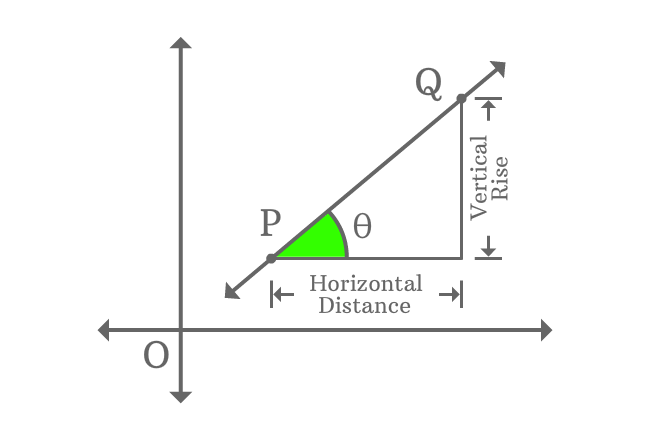# Slope of a Straight Line

The ratio between vertical rise of any two points on the line and horizontal distance between them is called slope of a straight line. It is also called as gradient of a straight line.

## IntroductionStraight lines are often appeared in Cartesian coordinate system with some inclination and it represents the slope of a straight line. It is essential to find the gradient of a straight line in geometric system. So, learn how to find the slope of a straight line in geometric methods firstly to study straight lines in geometry further.

The slope (or) gradient of a straight line is usually denoted by letter $m$ in geometric mathematics.

### Methods

There are two ways to find the gradient of a straight line in geometric mathematics.

#### Trigonometric form

The tangent of inclination of a straight line is slope of a straight line.

#### Coordinates form

The ratio of difference of ordinates of any two points on a line to difference of their abscissae is also gradient of straight line.

Latest Math Topics
Jun 26, 2023
Jun 23, 2023

Latest Math Problems
Jul 01, 2023
Jun 25, 2023
###### Math Questions

The math problems with solutions to learn how to solve a problem.

Learn solutions

Practice now

###### Math Videos

The math videos tutorials with visual graphics to learn every concept.

Watch now

###### Subscribe us

Get the latest math updates from the Math Doubts by subscribing us.